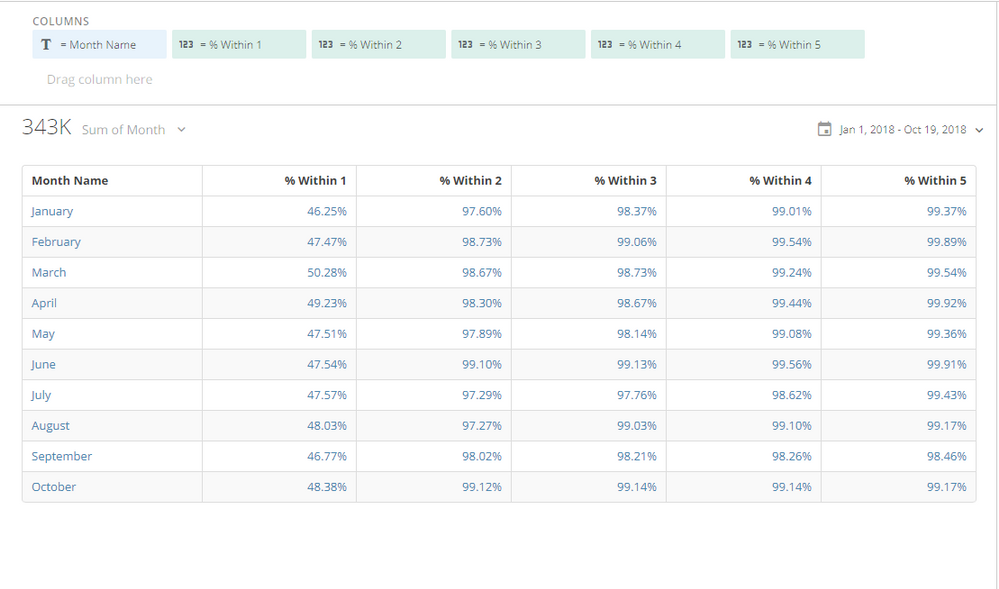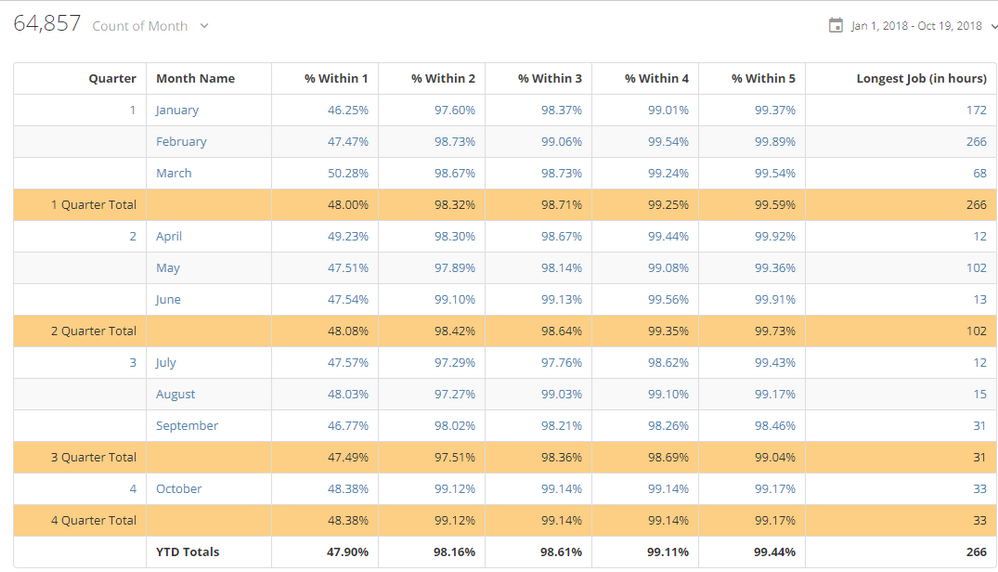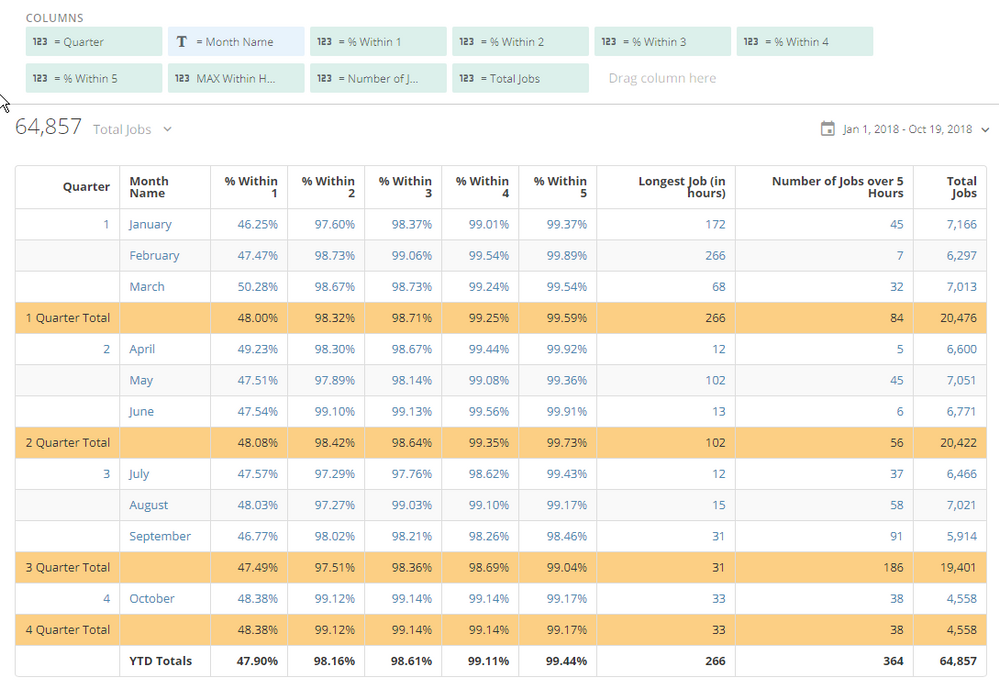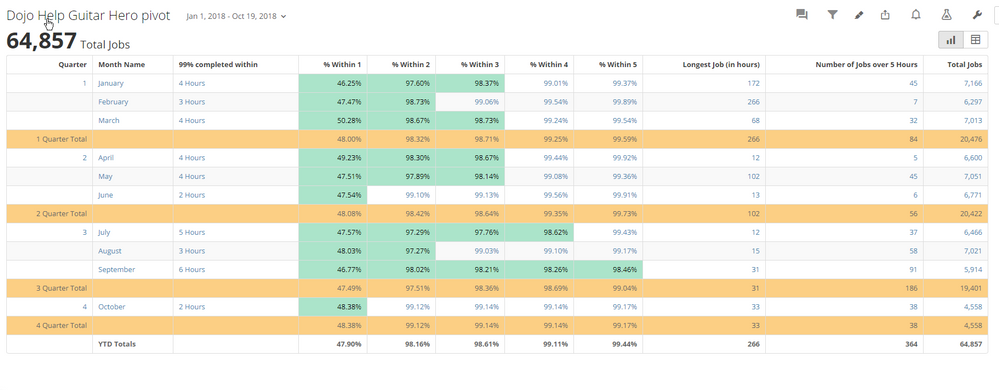# Recreating Excel's Pivot Chart "Show values as % Running Total In"

I'm trying to recreate this Excel Pivot Table in Domo (Currently as a Heat Map but if someone has a better suggestion I'm all ears).

(See "Domo - Pivot 1.JPG)

The good or bad thing is I can select the data from the database however I want so I'm free to do calculations before the data gets to Domo but the basics that make up this chart are

Within Hours: A CEILING value of the amount of time it took to complete a task. So if a task took 1.2 hours "Within Hours" would = 2

Month: A number representing a month value (January = 1, Feburary = 2). I'm thinking I can just use the full date field and group by month via the date picker in the card but the former is how it is in Excel.

Count of Date: This is simply doing a count of records, any field could be used. However this count is being represented as the % of the running total of the "Within Hours" field.

So you can read the chart as in January (1) 46.25% of tasks are being completed within 1 hour, 97.6% are completed within 2 hours and so on.

The calculation that we don't see that Excel is doing is the total row count for January = 7207. It's then taking the count of rows where Within Hours = 1 (3314) and dividing it against the total (7207) giving us 46.25%. Then for Within Hours = 2 it's taking the count of all rows where Within Hours = 1 or 2 (6993) and dividing against the total (7207). This tells us that 97.6% of tasks are done within two hours.

I've been able to get it to the # of tasks complete during each "Within Hours" so it shows as

January

Within 1 hour = 3314

Within 2 hours = 3679

Within 3 hours = 55

But what I need is

Within 1 hour = 3314 (shown as % compared to the total 7207

Within 2 hours = 6993 ( Within 1 + Within 2 as % compared to total 7207)

etc.

I have attached the data and pivot table from the report I get. See "Domo Test Data.xlsx".

• Does the Pivot table need to be set up that way?  Or can we swap the columns and rows?

I was able to get this with a few beastmodes:Month Name is just

MONTHNAME(`Date`)

and then % Within X is just

sum(Case when `Within Hours`<=X then 1 else 0 end) / count(`Date`)

you can change the X to 1,2,3,4,...n

• That's a good workaround that I'll fall back on thanks! My concern is that i technically could be any amount of hours so the beastmode would be very long. As of now 266 is the largest hours any takes which means at least 266 beast modes and a really long (horizontal) chart. Ideally it could be something more dynamic and preferably with the Within Hours being stacked vertically instead.

If it ends up not being possible maybe I can see if they'll be OK with bucketed values after a certain point so split out everything up until maybe hour 10 and then go 11-15. 16-20, etc or maybe they'll be OK with just splitting out the first 10 and then doing 10+.

Thanks for the help, definite progress and potential solution.

• wow, so you need to show all months to 100%?

Would it be worthwhile to have a final column that shows what the max hours to complete was?  Something like this:Or does the granularity need to be there, all the way to 266?

• I'll go back to the requester and ask if they need to get that specific. It may be a case of "That's how it was originally done" but not really necessary. Thanks for the help.

• Sure.  You may want to think about adding a few more columns to provide some additional information.  I think it would be most useful to understand what question they are wanting to answer with this table.

I added a few more columns that may provide some of the granularity they are looking for.  As you mentioned, maybe the cutoff they are interested in is 10 hours.• Based on the pivot chart you provided.  It looks like they are interested in understanding how many hours it took for 99% of the jobs to be completed.  Here is my final go at earning a solution here:• What did you put the colors on to get it like that? I had tried the same previously but it was just marking everything as colored, felt like a bug or an issue with how the data was structured.

This chart is basically an SLA to the customer as to how long we finish things in for them and we appear to color anything under 99%

Also what did you end up doing for the 99% complete within X hours beastmode?

• Nevermind....I stupidly left the apply to table row checkbox enabled. My question about the other column I'm still curious of

• There may be a more sophisticated way of writing this, but here is the beastmode I used:

case
when (sum(Case when `Within Hours`<=1 then 1 else 0 end) / count(`Date`)) >=.99 then '1 Hour'
when (sum(Case when `Within Hours`<=2 then 1 else 0 end) / count(`Date`)) >=.99 then '2 Hours'
when (sum(Case when `Within Hours`<=3 then 1 else 0 end) / count(`Date`)) >=.99 then '3 Hours'
when (sum(Case when `Within Hours`<=4 then 1 else 0 end) / count(`Date`)) >=.99 then '4 Hours'
when (sum(Case when `Within Hours`<=5 then 1 else 0 end) / count(`Date`)) >=.99 then '5 Hours'
when (sum(Case when `Within Hours`<=6 then 1 else 0 end) / count(`Date`)) >=.99 then '6 Hours'
else '7 or More Hours'
end

In your example, there weren't any months where the 99% cutoff was over 6 hours so I stopped there.

• Thanks you rock!